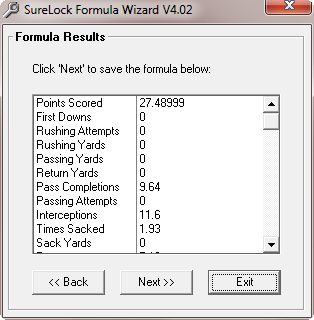## Section 11.1. Starting SureLock

To start SureLock, select SURELOCK FORMULA WIZARD from the ADD-INS menu. You'll see a screen like the one in Figure 11.10:Figure 11.10. The "Welcome to SureLock!" Screen

Select NEXT to move to the next screen. The screens will lead you through the steps required to create a prediction formula. Each step is explained in detail here.

To get the best results from SureLock, you may want to follow the steps in this section and create a new prediction formula during each week of the season. By doing so, you will always be using a formula derived from the latest data available, and this should increase your winning percentage.

### Step 1. Set the Sample Size

The sample size is simply the number of weeks of data to use when designing a formula. SureLock loads the data from these weeks and then builds a formula by correlating the data to actual game results.

You can select a sample size from 1 to 17 weeks. The actual data sample used depends upon the current date setting shown on the left side of the toolbar. For example, if you select a sample size of 8 weeks, the 8 weeks of data prior to the current date will be used to design the formula.

After selecting a sample size, select NEXT to proceed to the next step.

### Step 2. Automatic or Manual Stat Selection

SureLock can build a formula using stats that it selects, or stats that you select. If you want to pick the stats that will be included in the formula, select MANUAL MODE. If you want SureLock to pick the stats, select AUTOMATIC MODE.

If you select MANUAL MODE:

• A menu appears listing all of the stats that can be used in a prediction formula. Each stat has a check box that you can click on with your mouse. To deselect a stat, click on the box again. You can select a maximum of 10 stats. If 10 stats have already been selected, SureLock won't let you select any more. Please note that using more stats increases the time required to create a formula, and may not result in more accuracy. Usually, six stats are enough to tune a formula to beat the point spread. You should also note that some selected stats may not be considered important enough to be used in the formula.

If you select AUTOMATIC MODE:

• The AUTOMATIC STAT SELECTION menu appears. This menu allows you to pick the number of stats that you want to use in the prediction formula. Select a value from 1 to 10. SureLock will automatically determine the best stats to use based on how well they correlate to actual game results. Please note that using more stats increases the amount of time required to create a formula, and may not result in more accuracy. Usually, six stats are enough to tune a formula to beat the point spread. Also, some formulas may use one or two fewer statistics than you specified. This is because some data samples make it mathematically impossible to optimize the formula's percentage weights using the exact number of statistics that you specified.

When you finish selecting stats, select NEXT.

### Step 3. Select the Type of Formula

SureLock can create formulas that work best when recommending picks against the point spread (Sides), or when recommending over/under selections (Totals). At this stage, you need to decide whether you want a "Sides" formula or a "Totals" formula. After answering this question, select NEXT to continue.

At this stage, SureLock will create the formula. The FORMULA DESIGN IN PROGRESS screen will appear. As the formula is being built, the bar graphs on this screen will keep you informed of the progress.

Creating a formula involves many thousands of calculations, and SureLock may take a few seconds to build a formula on some computers. The time required to create a formula is roughly proportional to the sample size. For example, by using an 8 week sample (instead of 16 weeks) you could cut the times in half. However, the time required is not proportional to the number of weights. For example, using 10 weights may take three times longer than using 6 weights. If you need to, you can stop the process at any time by selecting EXIT. When the formula is completed, select NEXT.

### Step 5. Save the Formula

When the formula is finished, the formula window shown in Figure 11.11 will appear. The "Parameter" column shows the statistics that will be used by the formula. If you asked SureLock to pick the stats, they were chosen because they produced the highest correlation to the actual game results.Figure 11.11. The Formula Results Window

The "Value" column shows the percentage weight assigned to each stat, plus the optimum values to use for the home field advantage, the point spread multiplier, and the betting window. The sum of the percentage weights always equals 100.

When you are finished viewing the completed formula, select NEXT and select a name for the formula. Then, select NEXT again to save the formula. You may then create more formulas, or exit SureLock and return to PFA.